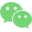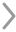WHCSRL 技术网

# [PyTorch Basic Course 7] Multi-dimensional feature input (I can’t learn to beat me)

## 学习总结

（1）y=Ax，矩阵A起到线性变换的作用，将x的N维度映射为M维度，即为一种空间变换的函数。而神经网络是想寻找一种非线性变换的空间函数，而可以通过多个线性变换层（下面栗子就是每次线性后加个非线性激活函数sigmoid），通过找到最优的权重，来组合起来，从而模拟非线性变换。
（2）relu取值范围也是0到1，但是如果input是小于0的则relu值为0（输出0是有风险的，因为后面可能会算ln 0，所以如果前面用的其他的激活函数，注意最后一个一般改为sigmoid激活函数，这样就能输出0到1之间数）。

## 一、和一维特征的区别sigmoid函数对向量中每个元素都sigmoid一次。ps：隐层越多，学习能力越强，但也不一定好，因为会学习到数据中的噪声，所以学习能力需要泛化能力。大学和高中的学习也是这样的思想，不需要死扣书本，而是需要学习读文档和基础架构的理念（泛化能力强）。

## 二、激活函数

relu取值范围也是0到1，但是如果input是小于0的则relu值为0（输出0是有风险的，因为后面可能会算ln 0，所以如果前面用的其他的激活函数，注意：最后一个一般改为sigmoid激活函数，这样就能输出0到1之间数）。## 三、糖尿病预测

``````# -*- coding: utf-8 -*-
"""
Created on Mon Oct 18 10:18:24 2021

@author: 86493
"""
import torch
import torch.nn as nn
import numpy as np
import matplotlib.pyplot as plt
# 这里的type不用double，特斯拉GPU才double
delimiter = ' ',
dtype = np.float32)

# 最后一列不要
x_data = torch.from_numpy(xy[: , : -1])
# [-1]则拿出来的是一个矩阵，去了中括号则拿出向量
y_data = torch.from_numpy(xy[:, [-1]])
losslst = []

class Model(nn.Module):
def __init__(self):
super(Model, self).__init__()
self.linear1 = nn.Linear(9, 6)
self.linear2 = nn.Linear(6, 4)
self.linear3 = nn.Linear(4, 1)
# 上次logistic是调用nn.functional的Sigmoid
self.sigmoid = nn.Sigmoid()
# 这个也是继承Module,没有参数,比上次写法不容易出错

def forward(self, x):
x = self.sigmoid(self.linear1(x))
x = self.sigmoid(self.linear2(x))
x = self.sigmoid(self.linear3(x))
return x

model = Model()

# 使用交叉熵作损失函数
criterion = nn.BCELoss(size_average = False)
optimizer = torch.optim.SGD(model.parameters(),
lr = 0.01)

for epoch in range(10):
y_predict = model(x_data)
loss = criterion(y_predict, y_data)
# 打印loss对象会自动调用__str__
print(epoch, loss.item())
losslst.append(loss.item())

# 梯度清零后反向传播
loss.backward()

# 更新权重
optimizer.step()

# 画图
plt.plot(range(10), losslst)
plt.ylabel('Loss')
plt.xlabel('epoch')
plt.show()
```123456789101112131415161718192021222324252627282930313233343536373839404142434445464748495051525354555657585960616263646566```## Reference

（1）PyTorch官方文档https://pytorch.org/docs/stable/_modules/torch
（2）b站视频：https://www.bilibili.com/video/BV1Y7411d7Ys?p=10
（3）吴恩达网易云课程：https://study.163.com/my#/smarts
（4）刘洪普老师博客：https://liuii.github.io/
（5）激活函数：http://rasbt.github.io/mlxtend/user_guide/general_concepts/activation-functions/#activation-functions-for-artificial-neural-networks
（6）激活函数演示：https://dashee87.github.io/data%%%%%%%%%%%%%%%%%%%%%%%%%%%%%%%%20science/deep%%%%%%%%%%%%%%%%%%%%%%%%%%%%%%%%20learning/visualising-activation-functions-in-neural-networks/
（7）pytorch官方文档，非线性激活函数：https://pytorch.org/docs/stable/nn.html#non-linear-activations-weighted-sum-nonlinearity微信公众号